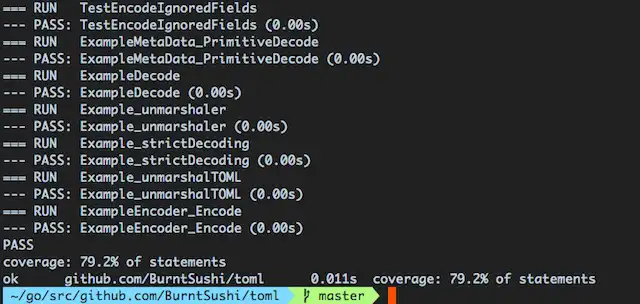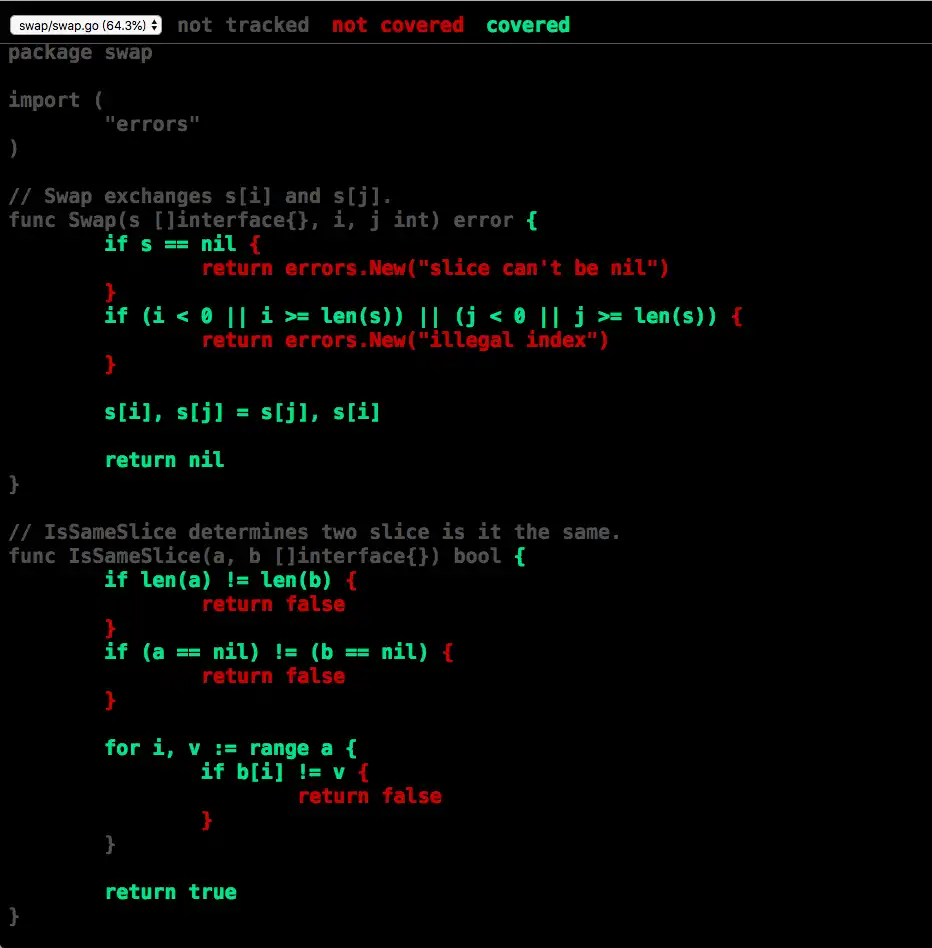## Go 语言对软件测试的支持

• testing 包：标准库中一个简单、强大的测试框架。
• go test 命令：`go tool` 中一个按照约定和组织的测试代码的命令。

### 规定（必须遵循的条例）：

• 只有以 `_test.go` 结尾的文件才会被视作是测试文件。如：`swap_test.go`
• 测试函数命名必须以 `Test` 开头，而后紧接着的第一个字母必须大写。如：`TestSwap`
• 测试函数必须传入一个指向 `testing.T``testing.B`（基准测试）的指针作为参数。如：`func TestSwap(t *testing.T)`，且没有返回值。

### 建议（最佳方法实践条例）：

• 将测试文件和要测试的代码放在同一个包（Go 语言组织也建议包名和文件所在目录名相同）。也就是说将这两个文件的 `package xxx` 写成相同的。
• 将测试文件命名为 `要测试的代码文件名_test.go`。如：`util.go` 的测试文件名应为 `util_test.go`
• 将测试函数命名为 `Test要测试的函数名称`。如：`Swap` 函数的测试函数命名应为 `TestSwap`，这个函数应只包含测试 `Swap` 函数的内容。

## Go 语言单元测试实践

### 示例函数说明

1. 文件名：`swap.go`
2. 包名：`swap`
3. 测试文件名：`swap_test.go`
4. 测试文件包名：`swap`

• 测试整个包: `go test swap``swap` 为基于 `\$GOPATH/src/` 的相对目录。
• 测试单个测试函数: `go test swap -run TestSwap``swap` 同样为相对目录，`TestSwap` 为测试函数名。

### 示例函数代码

 `````` 1 2 3 4 5 6 7 8 9 10 11 12 13 14 15 16 17 18 19 20 21 22 23 24 25 26 27 28 29 30 31 32 33 34 35 36 37 `````` ``````package swap import ( "errors" ) // Swap exchanges s[i] and s[j]. func Swap(s []interface{}, i, j int) error { if s == nil { return errors.New("slice can't be nil") } if (i < 0 || i >= len(s)) || (j < 0 || j >= len(s)) { return errors.New("illegal index") } s[i], s[j] = s[j], s[i] return nil } // IsSameSlice determines two slice is it the same. func IsSameSlice(a, b []interface{}) bool { if len(a) != len(b) { return false } if (a == nil) != (b == nil) { return false } for i, v := range a { if b[i] != v { return false } } return true }``````

### 单元测试示例

 `````` 1 2 3 4 5 6 7 8 9 10 11 12 13 14 15 16 17 18 19 20 21 22 23 24 25 26 27 28 29 30 31 `````` ``````package swap import ( "testing" ) func TestSwap(t *testing.T) { input1 := []interface{}{1, 2} i1 := 0 j1 := 1 want1 := []interface{}{2, 1} if err := Swap(input1, i1, j1); err != nil { t.Error(err) } if !IsSameSlice(input1, want1) { t.Errorf("got %v, want %v", input1, want1) } input2 := []interface{}{1, 'a', "aa"} i2 := 0 j2 := 2 want2 := []interface{}{"aa", 'a', 1} if err := Swap(input2, i2, j2); err != nil { t.Error(err) } if !IsSameSlice(input2, want2) { t.Errorf("got %v, want %v", input2, want2) } }``````

#### 表驱动测试示例

 `````` 1 2 3 4 5 6 7 8 9 10 11 12 13 14 15 16 17 18 19 20 21 22 23 24 25 26 `````` ``````package swap import ( "testing" ) func TestSwap(t *testing.T) { tests := []struct { input []interface{} i int j int want []interface{} }{ {[]interface{}{1, 2}, 0, 1, []interface{}{2, 1}}, {[]interface{}{1, 'a', "aa"}, 0, 2, []interface{}{"aa", 'a', 1}}, } for i, tt := range tests { if err := Swap(tt.input, tt.i, tt.j); err != nil { t.Error(err) } if !IsSameSlice(tt.input, tt.want) { t.Errorf("%v. got %v, want %v", i, tt.input, tt.want) } } }``````

### 查看测试覆盖率

• 测试整个包: `go test -cover=true swap`
• 测试单个测试函数: `go test -cover=true swap -run TestSwap`

`-cover=true` 选项会开启覆盖率说明

#### 生成 HTML 报告

`go test -cover=true swap -coverprofile=out.out` 将在当前目录生成覆盖率数据。## 第三方测试框架

### 使用 testify 包简化测试代码

`testify` 提供 `assert``mock``http` 三个包进行更多样的测试。下面用其中的 `assert` 包改写前面的例子。

 `````` 1 2 3 4 5 6 7 8 9 10 11 12 13 14 15 16 17 18 19 20 21 22 23 24 25 26 27 28 `````` ``````package swap import ( "github.com/stretchr/testify/assert" "testing" ) func TestSwap(t *testing.T) { input1 := []interface{}{1, 2} i1 := 0 j1 := 1 want1 := []interface{}{2, 1} if assert.Nil(t, Swap(input1, i1, j1)) { t.FailNow() } assert.Equal(t, input1, want1) input2 := []interface{}{1, 'a', "aa"} i2 := 0 j2 := 2 want2 := []interface{}{"aa", 'a', 1} if assert.Nil(t, Swap(input2, i2, j2)) { t.FailNow() } assert.Equal(t, input2, want2) }``````

### 使用 gotests 生成测试代码

`gotests` 可以为程序生成表驱动测试，在生成之后再添加测试数据即可完成测试代码的编写。

 `````` 1 2 3 4 5 6 7 8 9 10 11 12 13 14 15 16 17 18 19 20 21 22 23 24 25 `````` ``````package swap import "testing" func TestSwap(t *testing.T) { type args struct { s []interface{} i int j int } tests := []struct { name string args args wantErr bool }{ // TODO: Add test cases. } for _, tt := range tests { t.Run(tt.name, func(t *testing.T) { if err := Swap(tt.args.s, tt.args.i, tt.args.j); (err != nil) != tt.wantErr { t.Errorf("Swap() error = %v, wantErr %v", err, tt.wantErr) } }) } }``````

 `````` 1 2 3 4 5 6 7 8 9 10 11 12 13 14 15 16 17 18 19 20 21 22 23 24 25 26 `````` ``````package swap import "testing" func TestSwap(t *testing.T) { type args struct { s []interface{} i int j int } tests := []struct { name string args args wantErr bool }{ {"1", args{[]interface{}{1, 2}, 0, 1}, false}, {"2", args{[]interface{}{1, 'a', "aa"}, 0, 2}, false}, } for _, tt := range tests { t.Run(tt.name, func(t *testing.T) { if err := Swap(tt.args.s, tt.args.i, tt.args.j); (err != nil) != tt.wantErr { t.Errorf("Swap() error = %v, wantErr %v", err, tt.wantErr) } }) } }``````

## 参考链接

Go 单元测试

Go 语言实战笔记（二十一）| Go 单元测试